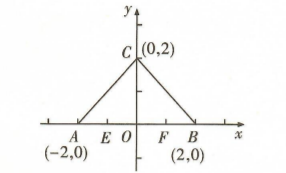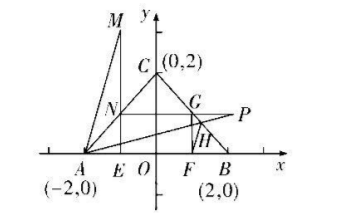$\text{A.}$ $(-\infty,-2)$ $\text{B.}$ $(0,+\infty)$ $\text{C.}$ $(1,+\infty)$ $\text{D.}$ $(4,+\infty)$
【答案】 D

【解析】 $\because A(-2,0), B(2,0), C(0,2), \therefore$ 直线 $B C$ 的方程为 $x+y-2=0$, 直线 $A C$ 的方程为 $x-y+2=0$, 如图, 作 $F$ 关于 $B C$ 的对称点 $P, \because F(1,0), \therefore P(2,1)$, 再作 $P$ 关于 $A C$ 的对称点 $M$, 则 $M(-1,4)$,$\because$ 直线 $F G$ 的方程为 $x=1$, 直线 $F H$ 的斜率为 $\frac{\frac{4}{5}}{\frac{6}{5}-1}=4, \therefore$ 直线 $F D$ 斜率的取值范围为 $(4,+\infty)$. 故选 D.